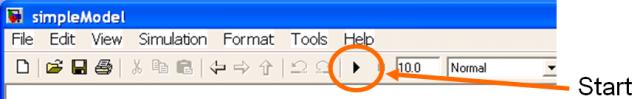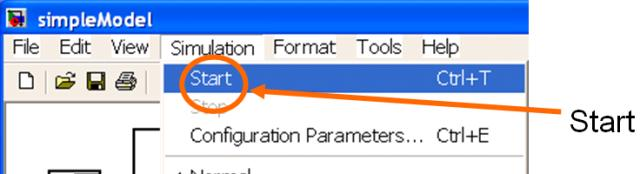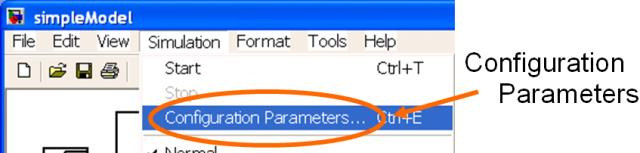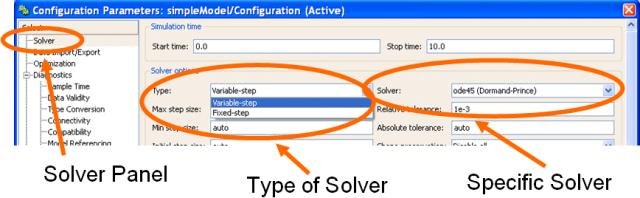This tutorial is the second in a series of tutorials that discuss the essential features of Simulink as required to model, simulate and analyse dynamic systems.

Specifically this tutorial covers topics related to setting the model parameters that determine how the simulation works. The topics are broken into the following sections,

See the Simulink Essentials - Building and Simulating Models page for links to tutorials discussing how to construct a model in Simulink. Other tutorials discussing Simulink and its applications for model based design are available on the Software Tutorials page.

### Starting a Simulation

There are two main approaches to starting simulations from a model’s user interface. The first is to press the Start button across the top of the model. This is the button that looks like a small black triangle pointing to the right and is shown in in Figure 1. (The triangle is designed to remind the user of the start button on a CD player.)Figure 1: Starting a Model Using the Start Button.

The second approach is to use the Simulation→Start Pull-Down Menu. This is shown in Figure 2. (Figure 2 also shows a third method, which is to use the Ctrl-T keyboard shortcut combination.)Figure 2: Starting a Model Using the Simulation→Start Pull-Down Menu.

Once the simulation is started the Start button changes to a Pause button. Pressing it pauses the simulation. It can be restarted (from the time that the button was pressed) by pressing the same button for a third time.

The simulation will run until the stop time is reached, or the Stop button is pressed, an an exception occurs which stops the simulation prematurely. Such an exception may be a divide by zero or some other anomaly that prevents the simulation from continuing.

Note that any data being saved to the MATLAB Workspace will only get written to MATLAB when the simulation is paused or stopped. That is, data is not written to MATLAB on a simulation time-step by time-step basis.

### Setting the Stop Time

The nominal simulation duration is specified by the model’s Stop Time parameter. This may be set to any positive number in the edit box across the top of the model’s user interface as shown in Figure 3.Figure 3: Specifying the Stop Time.

Note that time has no specific units in Simulink. It is purely a function of any time units used by parameters in the model. For instance, if the model uses signals measured in metres per second, and/or radians per second, then the Simulink time unit is naturally interpreted as seconds. Alternatively if the signals uses units of per hour or per day then the time unit would be hours or days respectively.

### Model and Solver Parameters

The simulation executes according to a set of Configuration Parameters. These parameters control how Simulink propogates the model, and particularly any continuous states, through time.

The Configuration Parameters are accessed from the Simulation→Configuration Parameters pull-down menu. This is shown in Figure 4Figure 4: How to Access the Configuration Parameters.

There are a vast number of parameters that control how a simulation gets executed. For the majority of them the default parameters are sufficient (at least most of the time) and they will not be discussed in this tutorial. However the interested reader is encouraged to read the Simulink documentation to understand what effect the various parameters have and what settings may be specified for them.

For this tutorial only a (small) subset of the Solver parameters need to be considered.Figure 5: The Solver Configuration Parameters.

Figure 5 shows the Solver panel of the Configuration Parameters. Of most importance (at least for the exposition here) is the selection of a suitable solver for the model constructed in the Model Building with Simulink tutorial, which is called simpleModel.

Solvers may be variable-step or fixed step, and each type has several different versions. For simpleModel the default variable-step ode45 solver is sufficient. However, the Max Step Size setting should be changed (from the default value of auto) to a numeric value of 0.01. (The value of 0.01 has been chosen somewhat randomly and any reasonably small value will suffice.)

Once the above change has been made the simulation may be run (see Starting a Simulation).

This tutorial has discussed topics related to executing (i.e. simulating) a Simulink model. The next tutorial in this series, Visualizing Simulation Results, discusses how to view the data generated by a Simulink model.